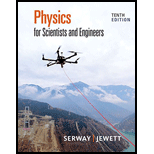Chapter 16, Problem 2PPhysics for Scientists and Enginee...

10th Edition
Raymond A. Serway + 1 other
ISBN: 9781337553278

Solutions

Chapter
SectionPhysics for Scientists and Enginee...

10th Edition
Raymond A. Serway + 1 other
ISBN: 9781337553278
Textbook Problem

Two points A and B on the surface of the Earth are at the same longitude and 60.0° apart in latitude as shown in Figure P16.2. Suppose an earthquake at point A creates a P wave that reaches point B by traveling straight through the body of the Earth at a constant speed of 7.80 km/s. The earthquake also radiates a Rayleigh wave that travels at 4.50 km/s. In addition to P and S waves. Rayleigh waves are a third type of seismic wave that travels along the surface of the Earth rather than through the bulk of the Earth. (a) Which of these two seismic waves arrives at B first? (b) What is the time difference between the arrivals of these two waves at B?Figure P16.2(a)

To determine

The wave which arrives first at the point B.

Explanation

The angle between the point A and B in latitude is 60.0°, the speed of P wave is 7.80km/s and the speed of Rayleigh wave is 4.50km/s.

P waves are the primary waves which are fastest among the other types of seismic waves

(b)

To determine

The time difference between the arrival of P wave and Rayleigh wave at the point B.

Still sussing out bartleby?

Check out a sample textbook solution.

See a sample solution

The Solution to Your Study Problems

Bartleby provides explanations to thousands of textbook problems written by our experts, many with advanced degrees!

Get Started

Find more solutions based on key concepts